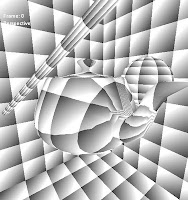## 02 November, 2010

### Stereoscopic test

Yesterday I did a small test with stereo rendering, trying to reproject the image from one eye to the other. It's very crude but I wanted to show the "theory" or the basic idea of the procedure as I got a few people asking me about it.Stereo rendering (standard red/cyan glasses needed)

Of course the most interesting thing is how to correctly inpaint the holes in your visibility. This is a very general problem that also applies to other post effects like motion blur and depth of field blur, the solution I use in this test well... I won't even call it a solution.

Much better methods have to be used for this to work decently, but that will (might...) be the topic of another post!Reconstructed viewRendered view

float Script : STANDARDSGLOBAL <
string UIWidget = "none";
string ScriptClass = "scene";
string ScriptOrder = "standard";
string ScriptOutput = "color";
string Script = "Technique=Main;";
> = 0.8; // version #

// -- Untweakables

float4x4 WorldViewProj : WorldViewProjection < string UIWidget="None"; >;
float4x4 WorldView : WorldView < string UIWidget="None"; >;
float4x4 Proj : Projection ;

float2 BufferSize : ViewportPixelSize < string UIWidget="None"; >;

float4 ClearParam < string UIWidget="None"; > = {0,0,0,0};

// -- Buffers and samplers

texture Bake : RENDERCOLORTARGET
<
float2 ViewPortRatio = {1,1};
string Format = "A8B8G8R8";
string UIWidget = "None";
>;
sampler2D BakeSampler = sampler_state
{
texture = ;
MagFilter = Point;
MinFilter = Point;
MipFilter = Point;
};

// -- Data structures

struct GeomVS_Out
{
float4 Pos : POSITION;
float4 PosCopy : TEXCOORD0;
float2 UV : TEXCOORD2;
};

{
float4 Pos : POSITION;
float2 UV : TEXCOORD0;
};

GeomVS_Out GeomVS(float4 pos : POSITION, float2 uv : TEXCOORD0)
{
GeomVS_Out Out;

Out.Pos = mul( pos, WorldViewProj );
Out.PosCopy = Out.Pos;
Out.UV = uv;

return Out;
}

{
return In;
}

// FX Composer does not pass near and far planes via a semantic, we have to "extract" them
float2 GetPlanesFromDxProjection()
{
float near = -Proj / Proj;
float far = -Proj / (Proj - 1.0);

return float2(near, far);
}

float4 EncUnitRangeToRGBA8( float v )
{
float4 enc = float4(1.0, 255.0, 65025.0, 160581375.0) * v;

enc = frac(enc);
enc -= enc.yzww * float4(1.0/255.0, 1.0/255.0, 1.0/255.0, 0.0);

return enc;
}

float DecUnitRangeToRGBA8( float4 rgba )
{
return dot( rgba, float4(1.0, 1/255.0, 1/65025.0, 1/160581375.0) );
}

float4 BakePS(GeomVS_Out In) : COLOR
{
// 0 to 1 from near to far
float linearDepth = In.PosCopy.z / GetPlanesFromDxProjection().y;

float testColor = dot(frac(In.UV.xy * 10) ,0.5.xx) + 0.25;

return float4(EncUnitRangeToRGBA8(linearDepth).xyz, testColor);
}

float4 DecodeBake(float2 uv)
{
float4 sample = tex2D(BakeSampler, uv);
float linearZ = DecUnitRangeToRGBA8(float4(sample.xyz,0));

// back to viewspace...
float2 nearFar = GetPlanesFromDxProjection();
linearZ = linearZ * (nearFar.y-nearFar.x) + nearFar.x;

#define VIEWTOUVMULT (float2(Proj, -Proj) * 0.5)
float2 xy = ((uv - VIEWTOUVADD) * linearZ)/VIEWTOUVMULT;

return float4(xy, linearZ, sample.w); // view xyz, pattern
}

{
return float4(DecodeBake(In.UV).zzz / 5, 1);
return float4(DecodeBake(In.UV).www, 1);
}

float3 ReprojectView(float3 viewPos)
{
// Here we should go from the view position of the baked eye to the one of the other
// it can be pretty generic but it assumes the difference will be only along the
// screen X axis...

return viewPos + float3(0.2,0,0);
}

{
float reprojDepth = 9999;
float reprojColor = 0;

float nearestColor = 0;
float nearestDistance = 9999;

float4 decodedCenter = DecodeBake(In.UV); // xyz = view, w = test pattern

float4 projectedCenter = mul(float4(decodedCenter.xyz,1), Proj);
projectedCenter /= projectedCenter.w;

for(float i=-63; i<64; i++) // note: brute force = bad, just a test
{
float2 sampleUV = In.UV + float2(i / BufferSize.x, 0);

// we assume in this test that the "color" is baked in w
// also, we assume that the scene fills the entire screen,
// we draw something everywhere...
float4 decoded = DecodeBake(sampleUV);

float3 newView = ReprojectView(decoded.xyz);

float4 projectedNewView = mul(float4(newView,1), Proj);
projectedNewView /= projectedNewView.w;

float rowDist = abs(projectedNewView.x - projectedCenter.x);

// Check if the new sample covers our pixel
// note: al these tests can be made "fuzzy" by constructing
if(rowDist < (2.0 / BufferSize.x))
{
if(reprojDepth>newView.z)
{
reprojColor = decoded.w;
reprojDepth = newView.z;
}
}

// We'll use this to fill the holes if any
// note: this is a very crude inpaiting method, something
// way better should be used for the method to work decently
float heuristicDistance = rowDist*rowDist / newView.z;
if(heuristicDistance < nearestDistance)
{
nearestColor = decoded.w;
nearestDistance = heuristicDistance;
}
}

// If we didn't find anything, we pull something from thin air...
if(reprojColor==0) reprojColor = nearestColor;

//return float4(decodedCenter.www,1);
//return float4(reprojColor.xxx,1);
return float4(reprojColor,decodedCenter.ww,1);
}

// -- Techniques

technique Main
<
string Script =
"Pass=Bake;"
"Pass=FullScreen;"
;
>
{
pass Bake
<
string Script =
"RenderColorTarget0=Bake;"
"ClearSetColor=ClearParam;"
"Clear=Color;"
"Clear=Depth;"
"Draw=Geometry;";
>
{
ZEnable = true;
ZWriteEnable = true;

}

pass FullScreen
<
string Script =
"RenderColorTarget0=;"
"Draw=Buffer;";
>
{
ZEnable = false;
ZWriteEnable = false;